## Aspire's Library

A Place for Latest Exam wise Questions, Videos, Previous Year Papers,
Study Stuff for MCA Examinations

## NIMCET Previous Year Questions (PYQs)

#### NIMCET Vector PYQ

NIMCET PYQ
If $\vec{a}=\hat{i}-\hat{k}$, $\vec{b}=x\hat{i}+\hat{j}+(1-x)\hat{k}$ and $\vec{c}=y\hat{i}+x\hat{j}+(1+x-y)\hat{k}$, then $\begin{bmatrix}{\vec{a}} & {\vec{b}} & {\vec{c}}\end{bmatrix}$ depends on

NIMCET Previous Year PYQNIMCET NIMCET 2023 PYQ

#### Solution

NIMCET PYQ
If $\vec{a}, \vec{b}$ are unit vectors such that $2\vec{a}+\vec{b} =3$ then which of the following statement is true?

NIMCET Previous Year PYQNIMCET NIMCET 2023 PYQ

#### Solution

NIMCET PYQ
$\theta={\cos }^{-1}\Bigg{(}\frac{3}{\sqrt[]{10}}\Bigg{)}$ is the angle between $\vec{a}=\hat{i}-2x\hat{j}+2y\hat{k}$ & $\vec{b}=x\hat{i}+\hat{j}+y\hat{k}$ then possible values of (x,y) that lie on the locus

NIMCET Previous Year PYQNIMCET NIMCET 2023 PYQ

#### Solution

NIMCET PYQ
If a vector having magnitude of 5 units, makes equal angle with each of the three mutually perpendicular axes,then the sum of the magnitude of the projections on each of the axis is

NIMCET Previous Year PYQNIMCET NIMCET 2023 PYQ

#### Solution

NIMCET PYQ
The value of non-zero scalars α and  β such that for all vectors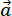and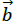such that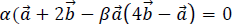is

NIMCET Previous Year PYQNIMCET NIMCET 2019 PYQ

#### Solution

NIMCET PYQ

A force of 78 grams acts at the point (2,3,5). The direction ratios of the line of action being 2,2,1 . The magnitude of its moment about the line joining the origin to the point (12,3,4) is

NIMCET Previous Year PYQNIMCET NIMCET 2019 PYQ

#### Solution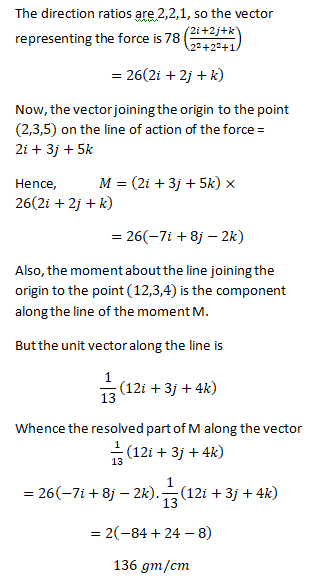NIMCET PYQ
The position vectors of the vertices

NIMCET Previous Year PYQNIMCET NIMCET 2019 PYQ

#### Solution

NIMCET PYQ

Not Available right now

NIMCET Previous Year PYQNIMCET NIMCET 2019 PYQ

#### Solution

|

NIMCET PYQ
Let $\vec{a}, \vec{b}, \vec{c}$ be distinct non-negative numbers. If the vectors $a\hat{i}+a\hat{j}+c\hat{k}$ , $\hat{i}+\hat{k}$ and $c\hat{i}+c\hat{j}+b\hat{k}$ lie in a plane, then c is

NIMCET Previous Year PYQNIMCET NIMCET 2022 PYQ

#### Solution

$\vec{a}=a\hat{i}+a\hat{j}+c\hat{k}\, ,\, \vec{b}=\hat{i}+\hat{k}\, \&\, \vec{c}=c\hat{i}+c\hat{j}+b\hat{k}$ are coplanar.

$\Rightarrow\begin{vmatrix}{a} & {a} & {c} \\ {1} & {0} & {1} \\ {c} & {c} & {b}\end{vmatrix}=0$

$\Rightarrow-ac-ab+ac+{c}^2=0$

$\Rightarrow{c}^2=ab$

NIMCET PYQ
If the volume of the parallelepiped whose adjacent edges are $\vec{a}=2\hat{i}+3\hat{j}+4\hat{k}$, $\vec{b}=\hat{i}+\alpha \hat{j}+2\hat{k}$ and $\vec{c}=\hat{i}+2\hat{j}+\alpha \hat{k}$ is 15, then $\alpha$ is equal to

NIMCET Previous Year PYQNIMCET NIMCET 2022 PYQ

#### Solution

NIMCET PYQ
Let $\vec{a}=2\hat{i}+2\hat{j}+\hat{k}$ and $\vec{b}$ be another vector such that $\vec{a}.\vec{b}=14$ and $\vec{a} \times \vec{b}=3\hat{i}+\hat{j}-8\hat{k}$ the vector $\vec{b}$ =

NIMCET Previous Year PYQNIMCET NIMCET 2022 PYQ

#### Solution

NIMCET PYQ
If $\vec{a}=\lambda \hat{i}+\hat{j}-2\hat{k}$ , $\vec{b}=\hat{i}+\lambda \hat{j}-2\hat{k}$ and $\vec{c}=\hat{i}+\hat{j}+\hat{k}$ and $\begin{bmatrix}{\vec{a}} & {\vec{b}} & {\vec{c}} \end{bmatrix}=7$, then the values of the $\lambda$ are

NIMCET Previous Year PYQNIMCET NIMCET 2022 PYQ

#### Solution

NIMCET PYQ
If $(\vec{a} \times \vec{b}) \times \vec{c}= \vec{a} \times (\vec{b} \times \vec{c})$, then

NIMCET Previous Year PYQNIMCET NIMCET 2022 PYQ

#### Solution

NIMCET PYQ
Let $\vec{a}=2\widehat{i}\, +\widehat{j}\, +2\widehat{k}$ , $\vec{b}=\widehat{i}-\widehat{j}+2\widehat{k}$ and $\vec{c}=\widehat{i}+\widehat{j}-2\widehat{k}$ are are three vectors. Then, a vector in the plane of $\vec{a}$ and $\vec{c}$ whose projection on $\vec{b}$ is of magnitude $\frac{1}{\sqrt{6}}$ is

NIMCET Previous Year PYQNIMCET NIMCET 2021 PYQ

#### Solution

NIMCET PYQ
If the position vector of A and B relative to O be $\widehat{i}\, -4\widehat{j}+3\widehat{k}$ and $-\widehat{i}\, +2\widehat{j}-\widehat{k}$ respectively, then the median through O of ΔABC is:

NIMCET Previous Year PYQNIMCET NIMCET 2021 PYQ

#### Solution

NIMCET PYQ
The area of the triangle formed by the vertices whose position vectors are $3\widehat{i}+\widehat{j}$ , $5\widehat{i}+2\widehat{j}+\widehat{k}$ , $\widehat{i}-2\widehat{j}+3\widehat{k}$ is

NIMCET Previous Year PYQNIMCET NIMCET 2021 PYQ

#### Solution

NIMCET PYQ
If the vectors $a\hat{i}+\hat{j}+\hat{k},\hat{i}+b\hat{j}+\hat{k},\hat{i}+\hat{j}+c\hat{k}$ , $(a,b,c\ne1)$ are coplanar, then $\frac{1}{1-a}+\frac{1}{1-b}+\frac{1}{1-c}=$

NIMCET Previous Year PYQNIMCET NIMCET 2021 PYQ

#### Solution

NIMCET PYQ
The direction cosines of the vector a = (- 2i + j – 5k) are

NIMCET Previous Year PYQNIMCET NIMCET 2017 PYQ

#### Solution

NIMCET PYQ
Let $\vec{a}=\hat{i}+\hat{j}$ and  $\vec{b}=2\hat{i}-\hat{k}$, the point of intersection of the lines $\vec{r}\times\vec{a}=\vec{b}\times\vec{a}$  and  $\vec{r}\times\vec{b}=\vec{a}\times\vec{b}$  is

NIMCET Previous Year PYQNIMCET NIMCET 2021 PYQ

#### Solution

NIMCET PYQ
If $\vec{a}$, $\vec{b}$ and $\vec{c}$ are vectors such that $\vec{a}$+$\vec{b}$+$\vec{c}$ = 0 and |$\vec{a}$| =7, $\vec{b}$=5,  |$\vec{c}$| = 3, then the angle between the vectors $\vec{b}$ and $\vec{c}$

NIMCET Previous Year PYQNIMCET NIMCET 2017 PYQ

#### Solution

NIMCET PYQ
If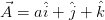,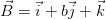and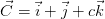, (a ≠ b ≠ c ≠ 1) are co-planar, then the value of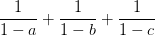is

NIMCET Previous Year PYQNIMCET NIMCET 2017 PYQ

#### Solution

NIMCET PYQ
Let a, b and c be three vectors having magnitudes 1, 1 and 2 respectively. If a x (a x c) - b = 0, then the acute angle between a and c is

NIMCET Previous Year PYQNIMCET NIMCET 2017 PYQ

#### Solution

NIMCET PYQ
Let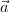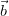and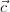be three vector such that || = 2, || = 3, || = 5 and++= 0. The value of.+.+.is

NIMCET Previous Year PYQNIMCET NIMCET 2017 PYQ

#### Solution

NIMCET PYQ
If,and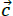are unit vectors, then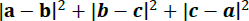does not exceeds

NIMCET Previous Year PYQNIMCET NIMCET 2018 PYQ

#### Solution

NIMCET PYQ
If= (i + 2j - 3k) and=(3i -j + 2k), then the angle between (+) and (-)

NIMCET Previous Year PYQNIMCET NIMCET 2017 PYQ

#### Solution

NIMCET PYQ
The vector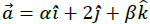lies in the plane of the vector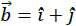and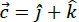and bisects the angle betweenand. Then which of the following gives possible values of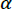and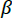?

NIMCET Previous Year PYQNIMCET NIMCET 2018 PYQ

#### Solution

NIMCET PYQ
A bird is flying in a straight line with velocity vector 10i+6j+k, measured in km/hr. If the starting point is (1,2,3), how much time does it to take to reach a point in space that is 13m high from the ground?

NIMCET Previous Year PYQNIMCET NIMCET 2018 PYQ

#### Solution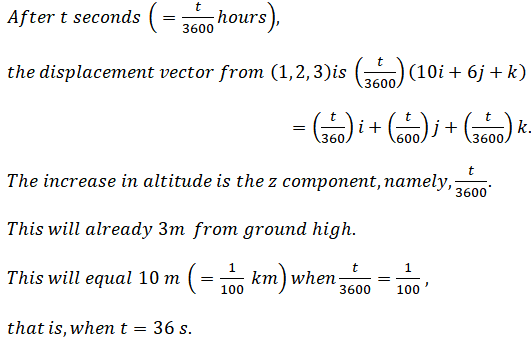NIMCET PYQ
Angle between $\vec{a}$ and  $\vec{b}$ is $120{^{\circ}}$. If $|\vec{b}|=2|\vec{a}|$ and the vectors , $\vec{a}+x\vec{b}$ ,   $\vec{a}-\vec{b}$ are at right angle, then $x=$

NIMCET Previous Year PYQNIMCET NIMCET 2021 PYQ

#### Solution

NIMCET PYQ
If $\vec{e_1}=(1,1,1)$ and $\vec{e_2}=(1,1,-1)$ and $\vec{a}$ and $\vec{b}$  and two vectors such that $\vec{e_2}=\vec{a}+2\vec{b}$ , then angle between $\vec{a}$ and $\vec{b}$

NIMCET Previous Year PYQNIMCET NIMCET 2021 PYQ

#### Solution

NIMCET PYQ
A cube is made up of 125 one cm. square cubes placed on a table. How many squares are visible only on three sides?

NIMCET Previous Year PYQNIMCET NIMCET 2017 PYQ

#### Solution

NIMCET PYQ
If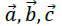are three non-coplanar vectors, then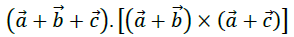NIMCET Previous Year PYQNIMCET NIMCET 2020 PYQ

#### Solution

NIMCET PYQ
Two forces F1 and F2 are used to pull a car, which met an accident. The angle between the two forces is θ . Find the values of θ for which the resultant force is equal to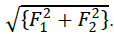NIMCET Previous Year PYQNIMCET NIMCET 2020 PYQ

#### Solution

NIMCET PYQ
If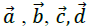are four vectors such that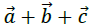is collinear with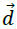and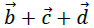is collinear with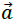then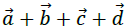=

NIMCET Previous Year PYQNIMCET NIMCET 2020 PYQ

#### Solution

NIMCET PYQ
Forces of magnitude 5, 3, 1 units act in the directions 6i + 2j + 3k, 3i - 2j + 6k, 2i - 3j - 6k respectively on a particle which is displaced from the point (2, −1, −3) to (5, −1, 1). The total work done by the force is

NIMCET Previous Year PYQNIMCET NIMCET 2020 PYQ

#### Solution

NIMCET PYQ
The position vectors of points A and B areand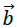. Then the position vector of point p dividing AB in the ratio m : n is

NIMCET Previous Year PYQNIMCET NIMCET 2020 PYQ

#### Solution

NIMCET PYQ
If a, b, c are three non-zero vectors with no two of which are collinear, a + 2b  is collinear with c and b + 3c is collinear with a , then | a + 2b + 6c | will be equal to

NIMCET Previous Year PYQNIMCET NIMCET 2020 PYQ

#### Solution

NIMCET PYQ
Vertices of the vectors i - 2j + 2k , 2i + j - k and 3i - j + 2k form a triangle. This triangle is

NIMCET Previous Year PYQNIMCET NIMCET 2020 PYQ

#### Solution

NIMCET PYQ
If the volume of a parallelepiped whose adjacent edges are
a = 2i + 3j + 4k,
b = i + αj + 2k
c = i + 2k + αk
is 15, then α =

NIMCET Previous Year PYQNIMCET NIMCET 2020 PYQ

#### Solution

NIMCET PYQ
If $\overrightarrow{{a}}$ and $\overrightarrow{{b}}$ are vectors in space, given by $\overrightarrow{{a}}=\frac{\hat{i}-2\hat{j}}{\sqrt[]{5}}$ and $\overrightarrow{{b}}=\frac{2\hat{i}+\hat{j}+3\hat{k}}{\sqrt[]{14}}$, then the value of$(2\vec{a} + \vec{b}).[(\vec{a} × \vec{b}) × (\vec{a} – 2\vec{b})]$ is

NIMCET Previous Year PYQNIMCET NIMCET 2023 PYQ

#### Solution

NIMCET PYQ
Let $\vec{A} = 2\hat{i} + \hat{j} – 2\hat{k}$ and $\vec{B} = \hat{i} + \hat{j}$, If $\vec{C}$ is a vector such that $|\vec{C} – \vec{A}| = 3$ and the angle between A × B and C is ${30^{\circ}}$, then $|(\vec{A} × \vec{B}) × \vec{C}|$ = 3 then the value of $\vec{A}.\vec{C}$ is equal to

NIMCET Previous Year PYQNIMCET NIMCET 2023 PYQ

#### NIMCET

Online Test Series,
and More.

#### NIMCET

Online Test Series,